# Stein Unbiased Risk Estimator¶

Important: Please read the installation page for details about how to install the toolboxes. $\newcommand{\dotp}{\langle #1, #2 \rangle}$ $\newcommand{\enscond}{\lbrace #1, #2 \rbrace}$ $\newcommand{\pd}{ \frac{ \partial #1}{\partial #2} }$ $\newcommand{\umin}{\underset{#1}{\min}\;}$ $\newcommand{\umax}{\underset{#1}{\max}\;}$ $\newcommand{\umin}{\underset{#1}{\min}\;}$ $\newcommand{\uargmin}{\underset{#1}{argmin}\;}$ $\newcommand{\norm}{\|#1\|}$ $\newcommand{\abs}{\left|#1\right|}$ $\newcommand{\choice}{ \left\{ \begin{array}{l} #1 \end{array} \right. }$ $\newcommand{\pa}{\left(#1\right)}$ $\newcommand{\diag}{{diag}\left( #1 \right)}$ $\newcommand{\qandq}{\quad\text{and}\quad}$ $\newcommand{\qwhereq}{\quad\text{where}\quad}$ $\newcommand{\qifq}{ \quad \text{if} \quad }$ $\newcommand{\qarrq}{ \quad \Longrightarrow \quad }$ $\newcommand{\ZZ}{\mathbb{Z}}$ $\newcommand{\CC}{\mathbb{C}}$ $\newcommand{\RR}{\mathbb{R}}$ $\newcommand{\EE}{\mathbb{E}}$ $\newcommand{\Zz}{\mathcal{Z}}$ $\newcommand{\Ww}{\mathcal{W}}$ $\newcommand{\Vv}{\mathcal{V}}$ $\newcommand{\Nn}{\mathcal{N}}$ $\newcommand{\NN}{\mathcal{N}}$ $\newcommand{\Hh}{\mathcal{H}}$ $\newcommand{\Bb}{\mathcal{B}}$ $\newcommand{\Ee}{\mathcal{E}}$ $\newcommand{\Cc}{\mathcal{C}}$ $\newcommand{\Gg}{\mathcal{G}}$ $\newcommand{\Ss}{\mathcal{S}}$ $\newcommand{\Pp}{\mathcal{P}}$ $\newcommand{\Ff}{\mathcal{F}}$ $\newcommand{\Xx}{\mathcal{X}}$ $\newcommand{\Mm}{\mathcal{M}}$ $\newcommand{\Ii}{\mathcal{I}}$ $\newcommand{\Dd}{\mathcal{D}}$ $\newcommand{\Ll}{\mathcal{L}}$ $\newcommand{\Tt}{\mathcal{T}}$ $\newcommand{\si}{\sigma}$ $\newcommand{\al}{\alpha}$ $\newcommand{\la}{\lambda}$ $\newcommand{\ga}{\gamma}$ $\newcommand{\Ga}{\Gamma}$ $\newcommand{\La}{\Lambda}$ $\newcommand{\si}{\sigma}$ $\newcommand{\Si}{\Sigma}$ $\newcommand{\be}{\beta}$ $\newcommand{\de}{\delta}$ $\newcommand{\De}{\Delta}$ $\newcommand{\phi}{\varphi}$ $\newcommand{\th}{\theta}$ $\newcommand{\om}{\omega}$ $\newcommand{\Om}{\Omega}$

This tour uses the Stein Unbiased Risk Estimator (SURE) to optimize the value of parameters in denoising algorithms.

In :
using PyPlot
using NtToolBox
# arequire("NtToolBox")


## Denoising and SURE¶

We consider a simple generative model of noisy images $F = f_0+W$ where $f_0 \in \RR^N$ is a deterministic image of $N$ pixels, and $W$ is a Gaussian white noise distributed according to $\Nn(0,\si^2 \text{Id}_N)$, where $\si^2$ is the variance of noise.

The goal of denoising is to define an estimator $h(F)$ of $f_0$ that depends only on $F$, where $h : \RR^N \rightarrow \RR^N$ is a potentially non-linear mapping.

Note that while $f_0$ is a deterministic image, both $F$ and $h(F)$ are random variables (hence the capital letters).

The goal of denoising is to reduce as much as possible the denoising error given some prior knowledge on the (unknown) image $f_0$. A mathematical way to measure this error is to bound the quadratic risk $\EE_W(\norm{h(F) - f_0}^2)$, where the expectation is computed with respect to the distribution of the noise $W$

For real life applications, one does not have access to the underlying image $f_0$. In this tour, we however assume that $f_0$ is known, and $f = f_0 + w\in \RR^N$ is generated using a single realization of the noise $w$ that is drawn from $W$. We define the estimated deterministic image as $h(f)$ which is a realization of the random vector $h(F)$.

Number $N = n \times n$ of pixels.

In :
n = 128*2
N = n^2;


First we load an image $f \in \RR^N$ where $N=n \times n$ is the number of pixels.

In :
f0 = load_image("NtToolBox/src/data/hibiscus.png", n);


Display it.

In :
figure(figsize = (5, 5))
imageplot(f0)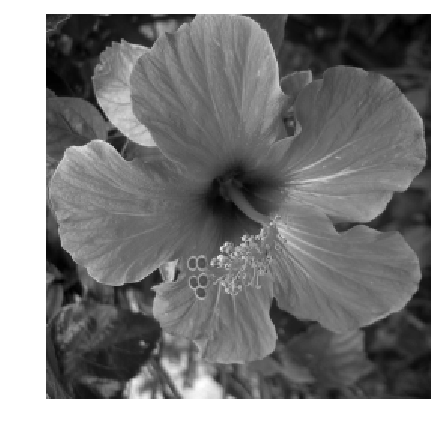Standard deviation $\si$ of the noise.

In :
sigma = .08;


Then we add Gaussian noise $w$ to obtain $f=f_0+w$.

In :
using Distributions
f = f0 .+ sigma.*rand(Normal(), n, n);


Display the noisy image. Note the use of the clamp function to force the result to be in $[0,1]$ to avoid a loss of contrast of the display.

In :
figure(figsize = (5, 5))
imageplot(clamP(f), @sprintf("Noisy, SNR = %.1f dB", snr(f0, f)));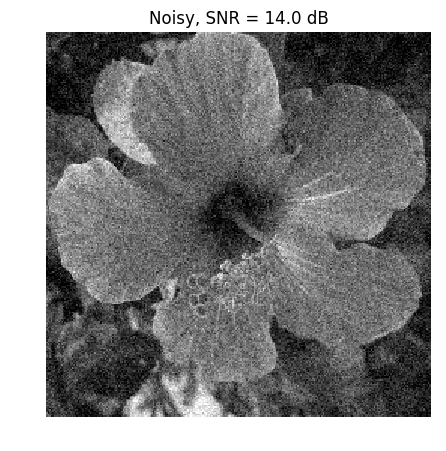The Stein Unbiased Risk Estimator (SURE) associated to the mapping $h$ is defined as

$$\text{SURE}(f) = -N\si^2 + \norm{h(f)-f}^2 + 2\si^2 \text{df}(f)$$

where df stands for degree of freedom, and is defined as

$$\text{df}(f) = \text{div} h(f) = \sum_i \pd{h}{f_i}(f).$$

It has been introduced in:

Stein, Charles M. (November 1981). "Estimation of the Mean of a Multivariate Normal Distribution". The Annals of Statistics 9 (6): 1135-1151.

And it has been applied to wavelet-based non-linear denoising in:

Donoho, David L.; Iain M. Johnstone (December 1995). "Adapting to Unknown Smoothness via Wavelet Shrinkage". Journal of the American Statistical Association (Journal of the American Statistical Association, Vol. 90, No. 432) 90 (432): 1200-1244.

If the mapping $f \mapsto h(f)$ is differentiable outside a set of zero measure (or more generally weakly differentiable), then SURE defines an unbiased estimate of the quadratic risk :

$$\EE_W(\text{SURE}(F)) = \EE_W( \norm{f_0-h(F)}^2 ).$$

This is especially useful, since the evaluation of SURE does not necessitate the knowledge of the clean signal $f_0$ (but note however that it requires the knowledge of the noise level $\si$).

In practice, one replaces $\text{SURE}(F)$ from its empirical evaluation $\text{SURE}(f)$ on a single realization $f$. One can then minimize $\text{SURE}(f)$ with respect to a parameter $\la$ that parameterizes the denoiser $h=h_\la$.

## Linear Denoising SURE¶

We consider a translation-invariant linear denoising operator, which is thus a convolution

$$h(f) = f \star g$$

where $g \in \RR^N$ is a low pass kernel, and $\star$ denotes the periodic 2-D convolution.

Since we use periodic boundary condition, we compute the convolution as a multiplication over the Fourier domain.

$$\forall \om, \quad \hat h(f)(\om) = \hat f(\om) \hat g(\om)$$

where $\hat g(\om)$ is the frequency $\om$ of the discrete 2-D Fourier transform of $g$ (computed using the pylab function fft2 from the pylab package).

In :
convol = (f, g) -> real(plan_ifft((plan_fft(f)*f).*(plan_fft(g)*g))
*((plan_fft(f)*f).*(plan_fft(g)*g)));


We define a parameteric kernel $g_\la$ parameterized by its bandwidth $\la>0$. We use here a Gaussian kernel

$$g_\la(a) = \frac{1}{Z_\la} e^{ -\frac{\norm{a}}{2 \la^2} }$$

where $Z_\la$ ensures that $\sum_a g_\la(a) = 1$.

In :
# include("NtToolBox/src/ndgrid.jl")
normalize = f -> f./sum(f)
x = [collect(0 : Base.div(n, 2)); collect(-Base.div(n, 2) + 1 : -1)]
(Y, X) = meshgrid(x, x)
g = lambd -> normalize(exp(-(X.^2 .+ Y.^2)/(2*lambd^2)));


Define our denoising operator $h=h_\la$ (we make explicit the dependency on $\la$): $$h_\la(f) = g_\la \star f.$$

In :
h = (f, lambd) -> convol(f, g(lambd));


Example of denoising result.

In :
lambd = 1.5

figure(figsize = (5, 5))
imageplot(clamP(h(f, lambd)))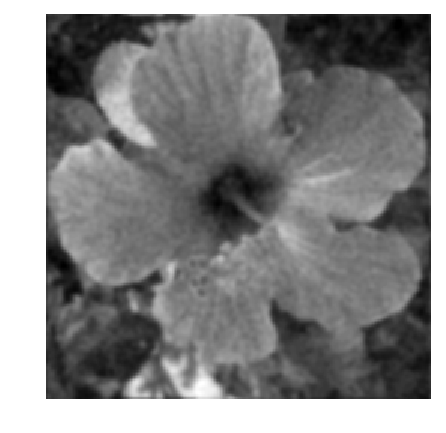For linear operator, the dregree of freedom is equal to the trace of the operator, and thus in our case it is equal to the sum of the Fourier transform $$\text{df}_\la(f) = \text{tr}(h_\la) = \sum_{\om} \hat g_\la(\om)$$ Note that we have made explicit the dependency of df with respect to $\la$. Note also that df$(f)$ actually not actually depend on $f$.

In :
df = lambd -> real(sum(plan_fft(g(lambd))*g(lambd)));


We can now define the SURE=SURE$_\la$ operator, as a function of $f, h(f), \lambda$.

In :
SURE = (f ,hf, lambd) -> -N*sigma^2 + vecnorm(hf - f)^2 + 2*sigma^2*df(lambd); # vecnorm is for Frobenius norm


Exercise 1

For a given $\lambda$, display the histogram of the repartition of the quadratic error $\norm{y-h(y)}^2$ and of $\text{SURE}(y)$. Compute these repartition using Monte-Carlo simulation (you need to generate lots of different realization of the noise $W$). Display in particular the location of the mean of these quantities.

In :
include("Exos/denoisingadv_9_sure/exo1.jl") #It takes time to run

could not open file /Users/gpeyre/Dropbox/github/numerical-tours/julia/Exos/denoisingadv_9_sure/exo1.jl

in include_from_node1(::String) at /Applications/Julia-0.5.app/Contents/Resources/julia/lib/julia/sys.dylib:?
in include_string(::String, ::String) at /Applications/Julia-0.5.app/Contents/Resources/julia/lib/julia/sys.dylib:?
In :
## Insert your code here.


In practice, the SURE is used to set up the value of $\la$ from a single realization $f=f_0+w$, by minimizing $\text{SURE}_\la(f)$.

Exercise 2

Compute, for a single realization $f=f_0+w$, the evolution of

$$E(\la) = \text{SURE}_\la(f) \qandq E_0(\lambda) = \norm{f-h_\la(f)}^2$$

as a function of $\lambda$.

In :
include("NtSolutions/denoisingadv_9_sure/exo2.jl")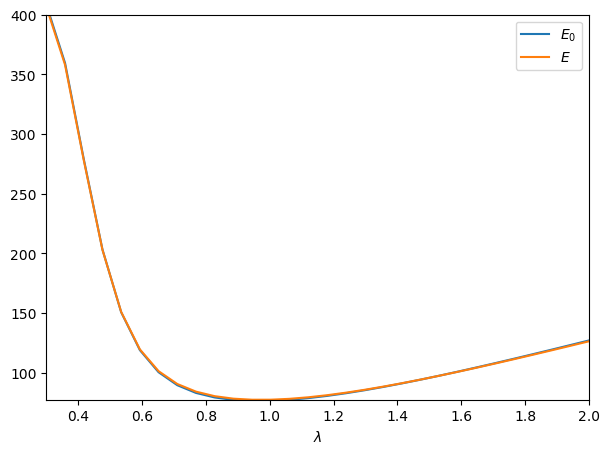In :
## Insert your code here.


Exercise 3

Display the best denoising result $h_{\la^*}(f)$ where $$\la^* = \uargmin{\la} \text{SURE}_\la(f)$$

In :
include("NtSolutions/denoisingadv_9_sure/exo3.jl")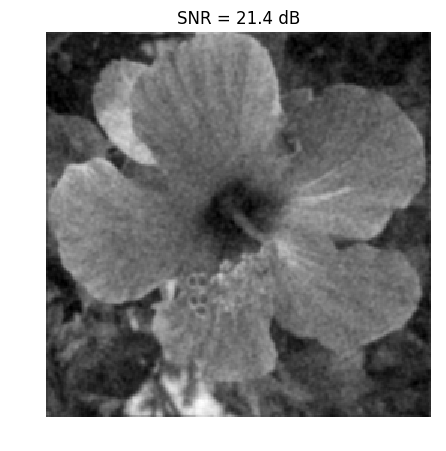Out:
PyObject <matplotlib.text.Text object at 0x33072dd50>
In :
## Insert your code here.


## Soft Thresholding SURE¶

In order to enhance the denoising results for piecewise regular signal and image, it is possible to use non-linear thresholding in an orthogonal wavelet basis $\Bb = \{ \psi_m \}_{m}$ where $\psi_m \in \RR^N$ is a wavelet element.

Re-generate a noisy image.

In :
f = f0 + sigma.*rand(Normal(), n, n);


The soft-thresholding estimator thus reads $$h_\la(f) = \sum_m s_\la( \dotp{f}{\psi_m} ) \psi_m \qwhereq s_\la(\al) = \max\pa{0, 1-\frac{\la}{\abs{\al}}} \al.$$ It can be conveniently written as $$h_\la = \Ww^* \circ S_\la \circ \Ww$$ where $\Ww$ and $\Ww^*$ are forward and inverse wavelet transform $$\Ww(f) = ( \dotp{f}{\psi_m} )_m \qandq \Ww^*(x) = \sum_m x_m \psi_m,$$ and $S_\la$ is the diagonal soft thresholding operator $$S_\la(x) = ( s_\la(x_m) )_m.$$

Define the wavelet transform and its inverse.

In :
h_daub = compute_wavelet_filter("Daubechies", 4)
W  = f1 -> NtToolBox.perform_wavortho_transf(f1,0,+1,h_daub)
Ws = x -> NtToolBox.perform_wavortho_transf(x,0,-1,h_daub);


Display the wavelet transform $\Ww(f_0)$ of the original image.

In :
figure(figsize = (10,10))
plot_wavelet(W(f0), 1)
show()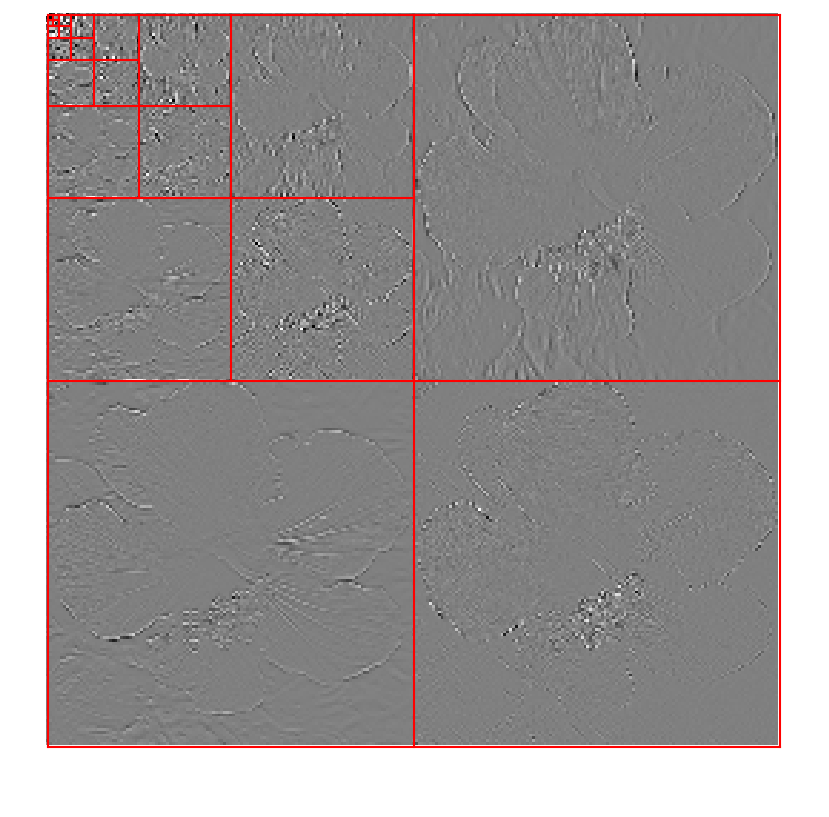Define the soft thresholding operator.

In :
S = (x, lambd) -> max(0, 1 - lambd./max(1e-9, abs(x)) ) .* x;


Define the denoising operator.

In :
h = (f1, lambd) -> Ws(S(W(f1), lambd));


Example of denoising result.

In :
lambd = 3*sigma/2

figure(figsize = (5, 5))
imageplot(clamP(h(f,lambd)))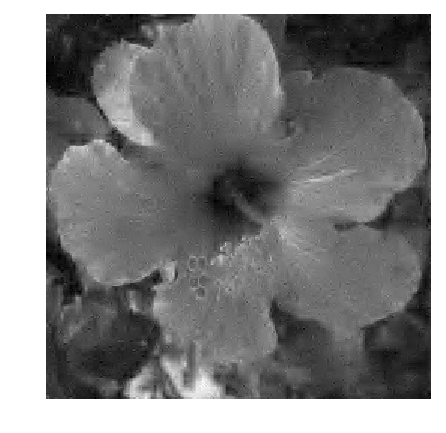Since $Ww$ is an orthogonal transform, one has $$\text{df}(f) = \text{div}( S_\la )( \Ww(f) ) = \sum_m s_\la'( \dotp{f}{\psi_m} ) = \norm{\Ww(h(f))}_0$$ where $s_\la'$ is the derivative of the 1-D function $s_\la$, and $\norm{\cdot}_0$ is the $\ell^0$ pseudo-norm $$\norm{x}_0 = \abs{ \enscond{m}{x_m \neq 0} }.$$

To summarize, the degree of freedom is equal to the number of non-zero coefficients in the wavelet coefficients of $h(f)$.

In :
df = (hf, lambd) -> sum(abs(W(hf)) .> 1e-8);


We can now define the SURE operator, as a function of $f, h(f), \lambda$.

In :
SURE = (f, hf, lambd) -> -N*sigma^2 + vecnorm(hf - f)^2 + 2*sigma^2*df(hf, lambd);


Exercise 4

For a given $\lambda$, display the histogram of the repartition of the quadratic error $\norm{y-h(y)}^2$ and of $\text{SURE}(y)$. Compute these repartition using Monte-Carlo simulation (you need to generate lots of different realization of the noise $W$). Display in particular the location of the mean of these quantities. Hint: you can do the computation directly over the wavelet domain, i.e. consider that the noise is added to the wavelet transform.

In :
include("NtSolutions/denoisingadv_9_sure/exo4.jl")

WARNING: hist(...) and hist!(...) are deprecated. Use fit(Histogram,...) in StatsBase.jl instead.
in depwarn(::String, ::Symbol) at ./deprecated.jl:64
in #hist!#994(::Bool, ::Function, ::Array{Int64,1}, ::Array{Any,1}, ::LinSpace{Float64}) at ./deprecated.jl:629
in hist(::Array{Any,1}, ::LinSpace{Float64}) at ./deprecated.jl:644
in include_from_node1(::String) at /Applications/Julia-0.5.app/Contents/Resources/julia/lib/julia/sys.dylib:?
in include_string(::String, ::String) at /Applications/Julia-0.5.app/Contents/Resources/julia/lib/julia/sys.dylib:?
in include_string(::Module, ::String, ::String) at /Users/gpeyre/.julia/v0.5/Compat/src/Compat.jl:577
in execute_request(::ZMQ.Socket, ::IJulia.Msg) at /Users/gpeyre/.julia/v0.5/IJulia/src/execute_request.jl:154
in invokelatest(::Function, ::ZMQ.Socket, ::Vararg{Any,N}) at /Users/gpeyre/.julia/v0.5/Compat/src/Compat.jl:587
in eventloop(::ZMQ.Socket) at /Users/gpeyre/.julia/v0.5/IJulia/src/eventloop.jl:8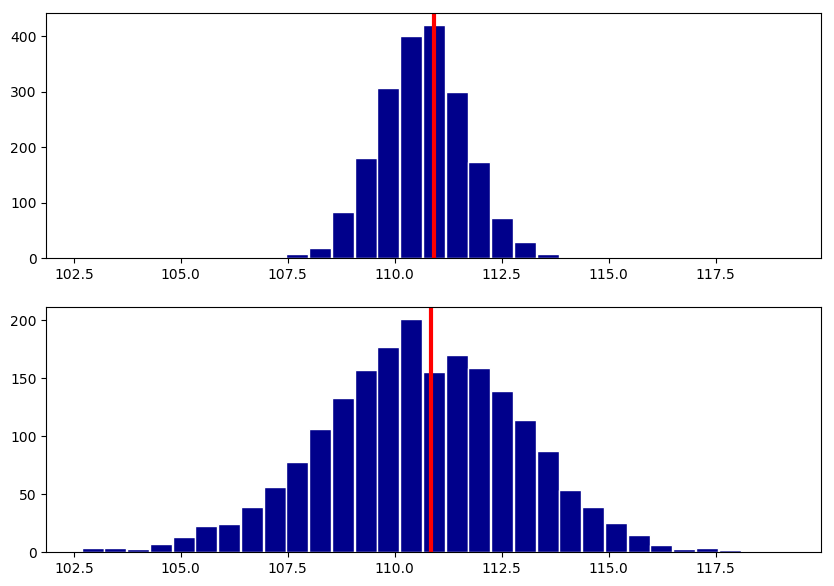WARNING: hist(...) and hist!(...) are deprecated. Use fit(Histogram,...) in StatsBase.jl instead.
in depwarn(::String, ::Symbol) at ./deprecated.jl:64
in #hist!#994(::Bool, ::Function, ::Array{Int64,1}, ::Array{Any,1}, ::LinSpace{Float64}) at ./deprecated.jl:629
in hist(::Array{Any,1}, ::LinSpace{Float64}) at ./deprecated.jl:644
in include_from_node1(::String) at /Applications/Julia-0.5.app/Contents/Resources/julia/lib/julia/sys.dylib:?
in include_string(::String, ::String) at /Applications/Julia-0.5.app/Contents/Resources/julia/lib/julia/sys.dylib:?
in include_string(::Module, ::String, ::String) at /Users/gpeyre/.julia/v0.5/Compat/src/Compat.jl:577
in execute_request(::ZMQ.Socket, ::IJulia.Msg) at /Users/gpeyre/.julia/v0.5/IJulia/src/execute_request.jl:154
in invokelatest(::Function, ::ZMQ.Socket, ::Vararg{Any,N}) at /Users/gpeyre/.julia/v0.5/Compat/src/Compat.jl:587
in eventloop(::ZMQ.Socket) at /Users/gpeyre/.julia/v0.5/IJulia/src/eventloop.jl:8

In :
## Insert your code here.


Exercise 5

Compute, for a single realization $f=f_0+w$, the evolution of

$$E(\la) = \text{SURE}_\la(f) \qandq E_0(\lambda) = \norm{f-h_\la(f)}^2$$

as a function of $\lambda$.

In :
include("NtSolutions/denoisingadv_9_sure/exo5.jl")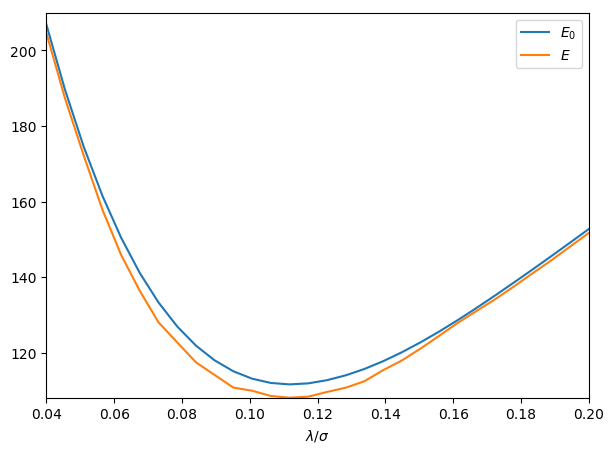In :
## Insert your code here.


Exercise 6

Display the best denoising result $h_{\la^*}(f)$ where $$\la^* = \uargmin{\la} \text{SURE}_\la(f)$$

In :
include("NtSolutions/denoisingadv_9_sure/exo6.jl")

SNR = 19